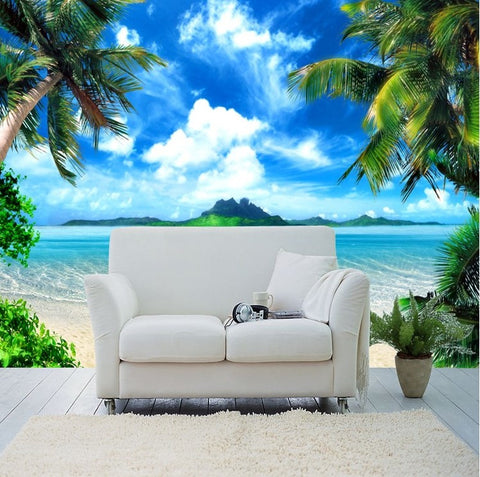## 3d Tropical Island Palm Trees Beach Scene Wallpaper

• \$49.95

Product Details

• Function: Waterproof, Sound-Absorbing, Heat Insulation, Smoke-Proof, Moisture-Proof, Mold-Proof, Soundproof, Anti-static
• Feature: Formaldehyde-free, Mildew Resistant, Extra Thick, Environment Friendly
• Surface Treatment: Embossed
• Style: Modern
• Pattern: Island beach

Please note: 1 square meter is \$49.95.  It is a small size.  The example pictures feature a fully covered wall.  This is not just 1 square meter.  You need to measure your wall and select how many square meters you need to purchase.  If you need help with calculations just let us know.  We would be happy to help you.

How to calculate how many meters you need:
Measure your wall.  For example, if your wall is 360cm width x 240cm height, add 5 to both sides:
(360+5) x (240+5) = 8.9cm² = 9cm².  So you would need to order 9 pieces (9 square meters).
Here are some fixed sizes:

Quantity 1 square meters  =  130cm (width) x 75cm (height) ( W 52inch x H 29inch )

Quantity 2 square meters  =  170cm (width) x115cm (height) ( W 67inch x H 46inch )

Quantity 3 square meters  =  200cm (width) x 150cm (height) (W 79inch x H 59inch )

Quantity 4 square meters  =  200cm (width) x 190cm (height) ( W 79inch x H 75inch )

Quantity 5 square meters    =    250cm (width) x 200cm (height) ( W 98inch x H 79inch )

Quantity 6 square meters    =    255cm (width) x 220cm (height) ( W 100inch x H 87inch )

Quantity 7 square meters    =    300cm (width) x 230cm (height) (W 118inch x H 90inch )

Quantity 8 square meters    =    340cm (width) x 230cm (height) ( W 134inch x H 90inch )

Quantity 9 square meters    =    400cm (width) x 220cm (height) ( W 157inch x H 87inch )

Quantity 10 square meters    = 400  cm (width) x 250cm (height) ( W 157inch x H 98inch )

Quantity 12 square meters    =    450cm (width) x 260cm (height) ( W 177inch x H 102inch )

Quantity 14 square meters    =    500 cm (width) x270cm (height) ( W 199inch x H 106inch )

We Also Recommend